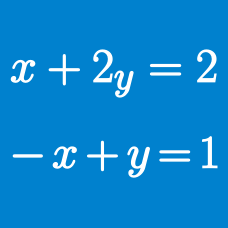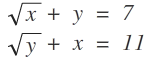Algebra

# System of Equations: Level 2 Challenges

$\begin{cases} { x }^{ 2 }+{ y }^{ 2 }=30 \\ x+y=10 \end{cases}$

If the above equations are true simultaneously, then find the value of $xy$.

$\large \begin{cases} { a(b+c)=32 } \\ { b(c+a) = 65 } \\ {c(a+b) = 77 } \end{cases}$

Given that $a , b,$ and $c$ are positive real numbers that satisfy the system of equations above, find the value of $abc$.

Given that ${ a }^{ 2 }+{ b }^{ 2 }={ c }^{ 2 }+{ d }^{ 2 }=1$ And also that $ac+bd=0$ What is the value of $ab+cd$?

For the positive numbers $d, o, v, e$: $d^2 + do = 250 \\ o^2 + do = 150$ $v^2 + ve = 30 \\ e^2 + ve = 34$ Find the value of $d + o + v + e$.You think you rock at linear equation? Gimme the solution NOT using hit and trial method! Solve for the values of "x" and "y" and enter $x+y$

Note: These are not linear equations.

×

Problem Loading...

Note Loading...

Set Loading...